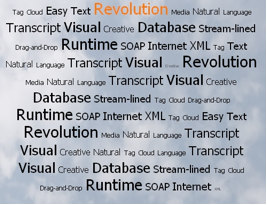Issue 51June 20, 2008Revolution in the Clouds
Creating tag clouds in Revolution

A couple of days ago I was discussing "Tag Clouds" with some colleagues and the conversation turned to "how easy would it be to do in Rev?". The answer, as I am sure you have already guessed, was "very". And so I present today's technical article - a simple Tag Cloud that can be used to visually represent a set of weighted words.Although this is not a proper "Tag Cloud" - it does not rearrange the words so the heaviest weighted are in the middle - it is a very fast, easy version that uses the field object to perform all the hard work.

We use the field object for the simple reason that every word in a field can have a different font size and that the field object will automatically wrap text to the next line if we tell it to. So all we need to do is create a long list of the words (without any returns) where each word is set to the font size that represents its weight and the field object will do the rest.

In this example I have also added some code to show mouse roll-over - i.e. each word changes color when you move the mouse over it - using the mouseChunk function.

The example application can be downloaded here.

OK - so lets get coding.

Firstly we need an algorithm that will calculate the correct font size for each word. This algorithm should set the heaviest weighted item to the maximum specified font size and the lightest weighted item to the minimum specified font size. All other words should be linearly scaled between these two values depending upon their weight.

We first need to calculate the maximum and the minimum weightings in the list. As we have a data field where each line is a (word,weight) pair we need to convert this into a comma separated list of just the weightings, then we can use the built in Revolution functions to calculate the max and min of the list. The function getValues() below does this.

```function getValues
local tNums, tData
put field 1 into tData
repeat for each line tLine in tData
put item 2 of tLine & comma after tNums
end repeat
return tNums
end getValues
```

We can use the return comma separated list of values that is returned by this function in Revolution's max() and min() functions.

The next thing we need to do at this point is set the textHeight of the field to a suitable value that will mean that lines of differing size text do not draw over each other. This is easy though as we are specifying what the maximum textSize is going to be, so we can just set the textHeight of the field to the maximum textSize we can possibly get - and then add in a little bit to space things out a touch:-

```set the textHeight of field "cloud" to tMaxPercent + 2
```

OK, so now we know the bounds ( i.e. Max and Min) of the weights in the dataset we can work out the scale that each data item should be set to. For each line in the data set we perform the following steps.

First we get the word and its weight:

```local tWord, tCount
put item 1 of tLine into tWord
put item 2 of tLine into tCount
```

Next, we can use all the information we have at this point to calculate the required size of the word based upon its weighting:

```put (tMaxPercent-tMinPercent)/(tMax-tMin) into tMulti
put tMinPercent + ((tMax-(tMax-(tCount-tMin)))*tMulti) into tSize
```

Now we have the textSize that is correct for the weight of the word in tSize.

The final thing to do is to add the word to the end of the field and then set the text size of the last word (which will be the one we have just added) to the size that was calculated using the code above.

```put space & tWord & space after field "cloud"
set the textSize of the last word of field "cloud" to tSize
```

Note: We add a space around the word we are adding so that Revolution will treat it as a separate word.

Now for the finishing touches to our tag cloud - roll over states. Revolution is so powerful when used to manipulate text data that to create a function that will highlight each word your mouse rolls over is a matter of a dozen lines of code - thanks to the mouseChunk function.

This function is simply a chunk expression that can be used in other statements to reference the word in a field under the mouse pointer.

To create the roll over code we keep a script local, sOldChunk, that references the last word that we were over. We then use the mouseMove handler to test if this chunk has changed - i.e. our mouse is now over a different word. If it is, we set the old word back to being black and set the new word to orange. Simple!

```on mouseMove pX, pY
if the mouseChunk <> sOldChunk then
if sOldChunk is not empty then
set the foregroundColor of sOldChunk to "black"
end if
if the mouseChunk is not empty then
set the foregroundColor of the mouseChunk to "orange"
put the mouseChunk into sOldChunk
end if
end if
end mouseMove
```

And that is all there is to it really - a nice and easy Revolution based "tag cloud". It's easily extended to be more "widget" like with the use of custom properties etc, but I hope this article gives you some ideas on novel ways to use the field object.Sneak Peek at the Next RevolutionRevolution in the CloudsAnimationEngine 2.9Revolution Beta Is Back